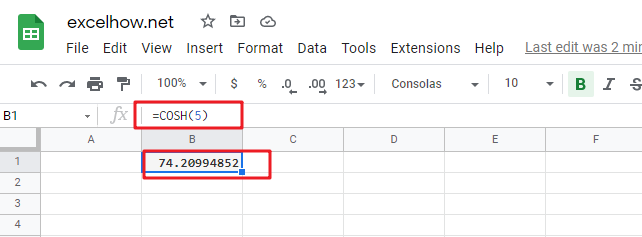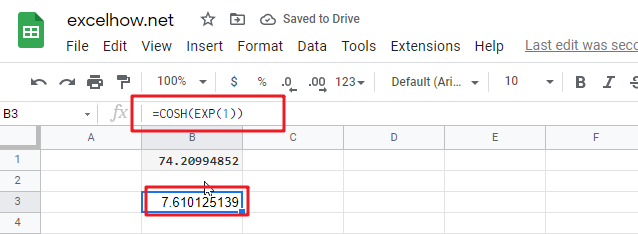# ExcelHow

This post will guide you how to use Google Sheets COSH function with syntax and examples.

## Description

The Google Sheets COSH function returns the hyperbolic cosine of a given angle.

The purpose of this function is used to get hyperbolic cosine of a number in google sheet and it’s returned value is the x-component of the hyperbolic angle.

The COSH function is a build-in function in Google Sheets and it is categorized as a MATH function.

## Syntax

The syntax of the COSH function is as below:

= COSH(number)

Where the COSH function argument is:

• numberThis is a required argument. Any real number that you want to calculate the hyperbolic cosine.

## Google Sheets COSH Function Examples

The below examples will show you how to use google sheets COSH Function to calculate the hyperbolic cosine of a supplied number.

1# get the hyperbolic cosine of 5, enter the following formula in Cell B1.

`=COSH(5)`2# get the hyperbolic of the base of the natural logarithm, enter the following formula in Cell B2.

`=COSH(EXP(1))`